Chapter Four Utility Utility Functions Indiff Curves u

• Slides: 30Chapter Four Utility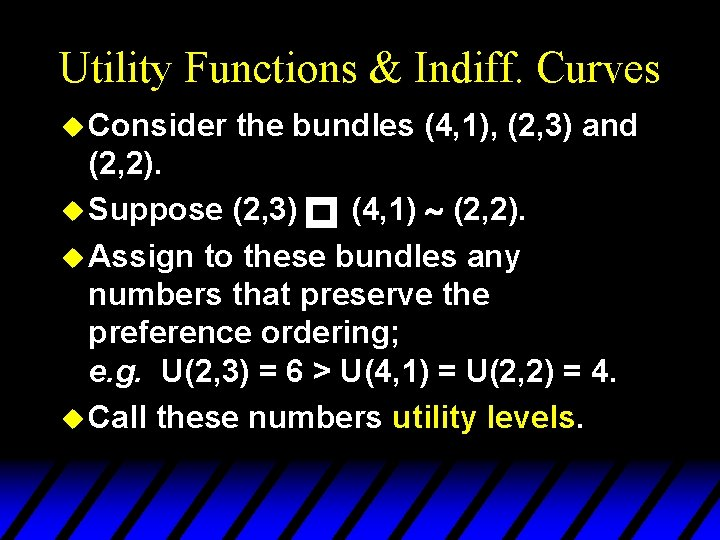Utility Functions & Indiff. Curves u Consider the bundles (4, 1), (2, 3) and (2, 2). u Suppose (2, 3) (4, 1) ~ (2, 2). u Assign to these bundles any numbers that preserve the preference ordering; e. g. U(2, 3) = 6 > U(4, 1) = U(2, 2) = 4. u Call these numbers utility levels. pUtility Functions & Indiff. Curves u An indifference curve contains equally preferred bundles. preference same utility level. u Therefore, all bundles in an indifference curve have the same utility level. u Equal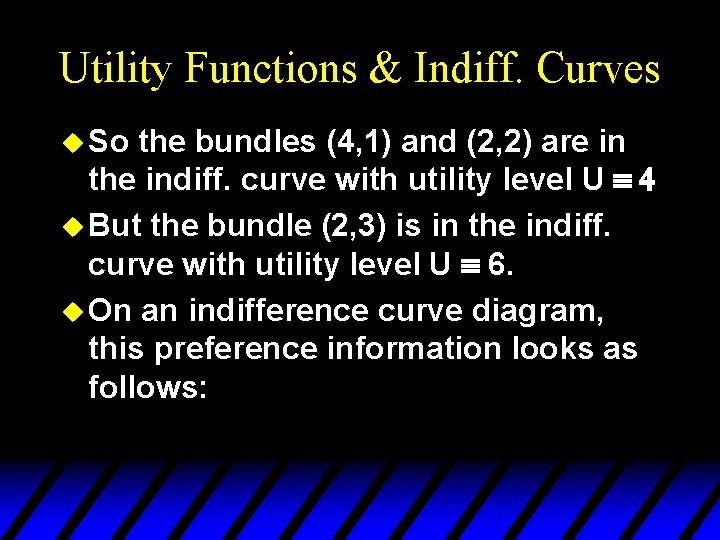Utility Functions & Indiff. Curves u So the bundles (4, 1) and (2, 2) are in the indiff. curve with utility level U º 4 u But the bundle (2, 3) is in the indiff. curve with utility level U º 6. u On an indifference curve diagram, this preference information looks as follows:Utility Functions & Indiff. Curves p x 2 (2, 3) (2, 2) ~ (4, 1) Uº 6 Uº 4 x 1Utility Functions & Indiff. Curves u Another way to visualize this same information is to plot the utility level on a vertical axis.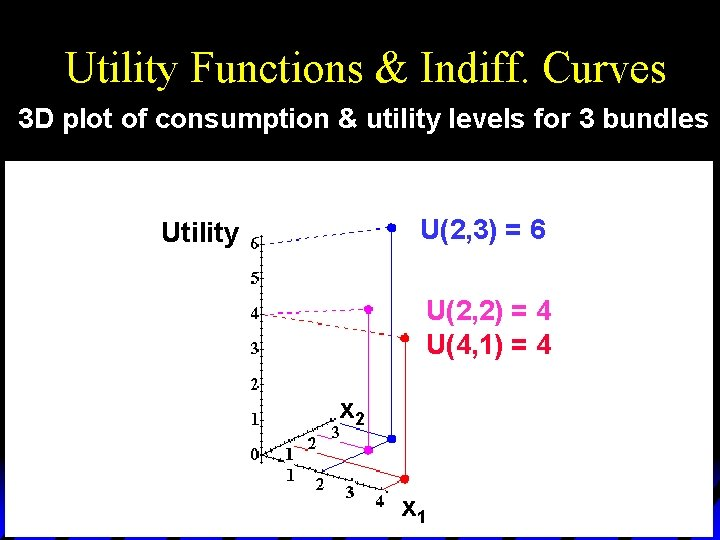Utility Functions & Indiff. Curves 3 D plot of consumption & utility levels for 3 bundles U(2, 3) = 6 Utility U(2, 2) = 4 U(4, 1) = 4 x 2 x 1Utility Functions & Indiff. Curves u This 3 D visualization of preferences can be made more informative by adding into it the two indifference curves.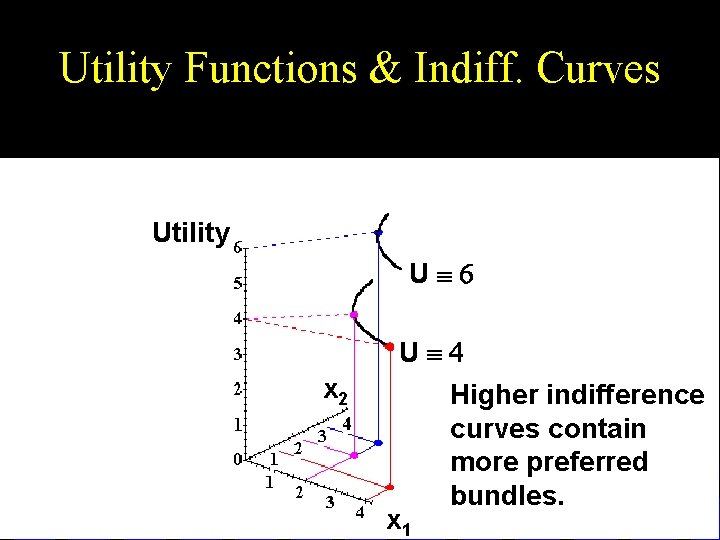Utility Functions & Indiff. Curves Utility Uº 6 Uº 4 x 2 x 1 Higher indifference curves contain more preferred bundles.Utility Functions & Indiff. Curves u Comparing more bundles will create a larger collection of all indifference curves and a better description of the consumer’s preferences.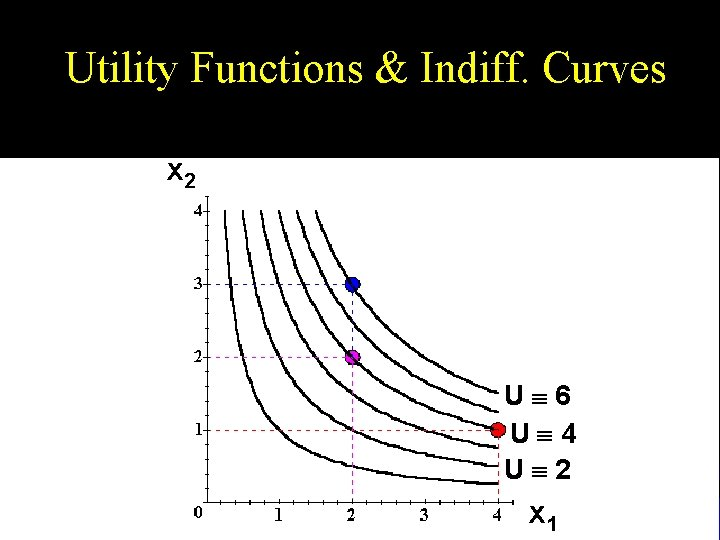Utility Functions & Indiff. Curves x 2 Uº 6 Uº 4 Uº 2 x 1Utility Functions & Indiff. Curves u As before, this can be visualized in 3 D by plotting each indifference curve at the height of its utility index.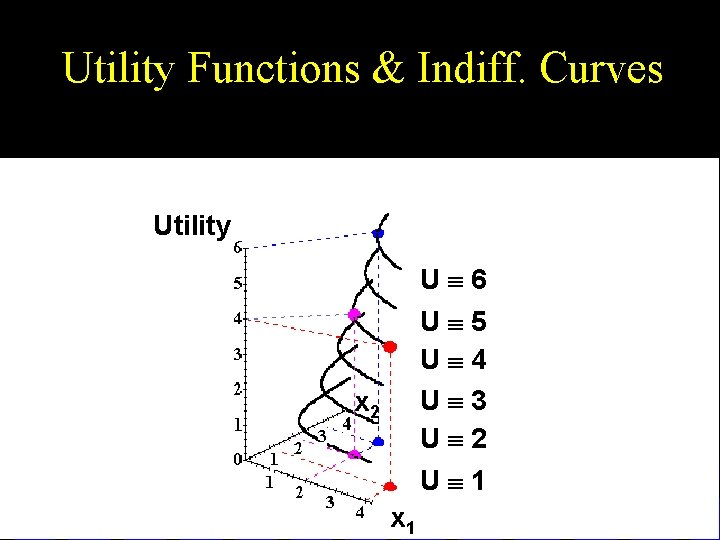Utility Functions & Indiff. Curves Utility Uº 6 Uº 5 Uº 4 Uº 3 Uº 2 x 2 Uº 1 x 1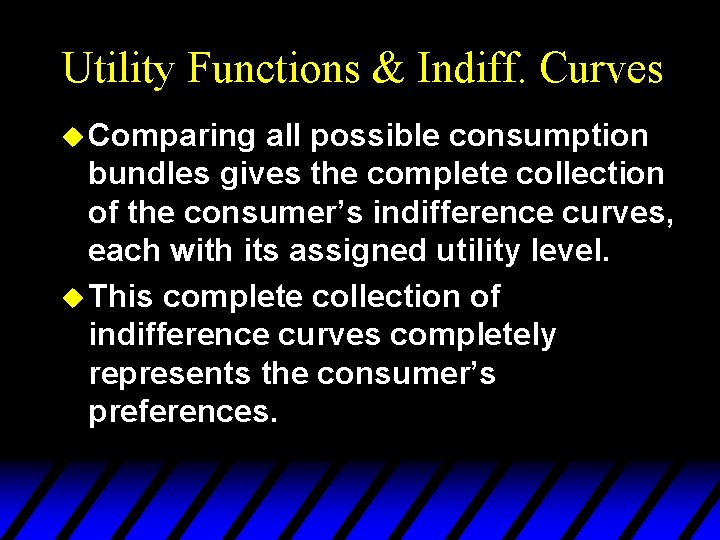Utility Functions & Indiff. Curves u Comparing all possible consumption bundles gives the complete collection of the consumer’s indifference curves, each with its assigned utility level. u This complete collection of indifference curves completely represents the consumer’s preferences.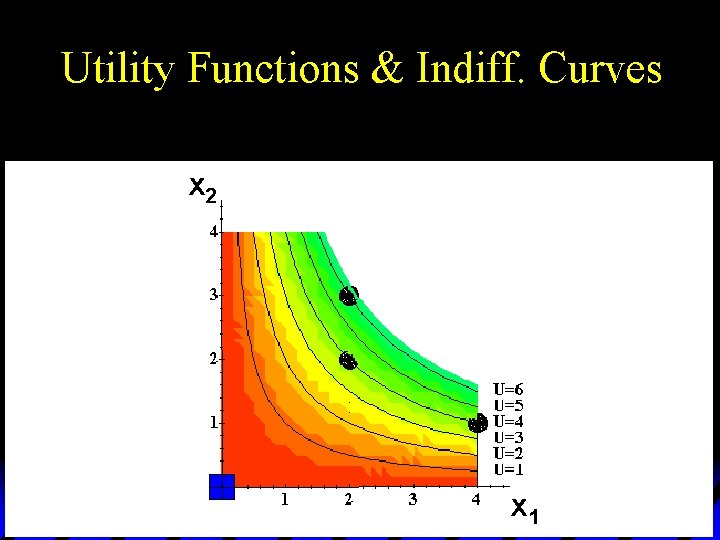Utility Functions & Indiff. Curves x 2 x 1Utility Functions & Indiff. Curves x 2 x 1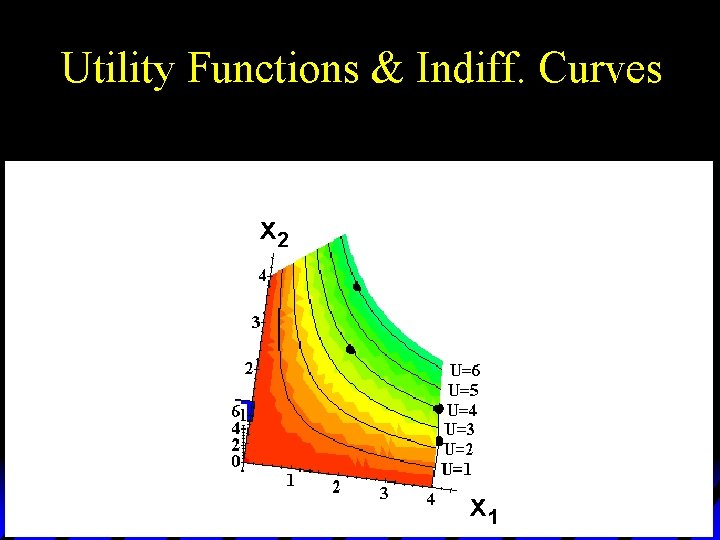Utility Functions & Indiff. Curves x 2 x 1Utility Functions & Indiff. Curves x 2 x 1Utility Functions & Indiff. Curves x 2 x 1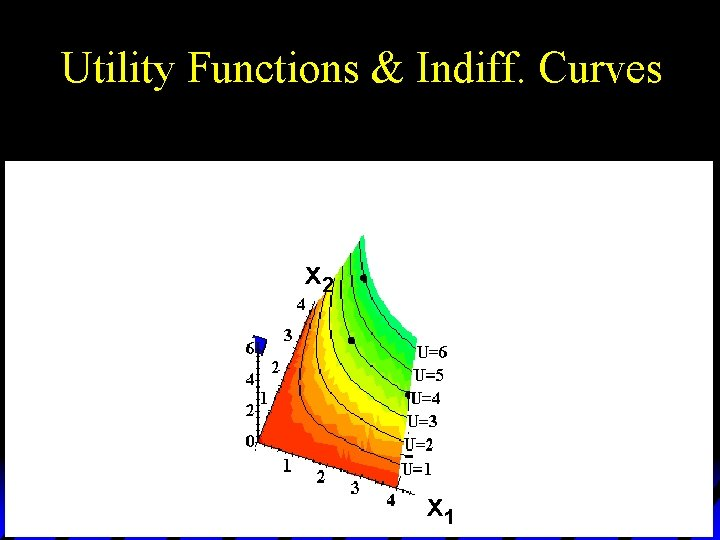Utility Functions & Indiff. Curves x 2 x 1Utility Functions & Indiff. Curves x 1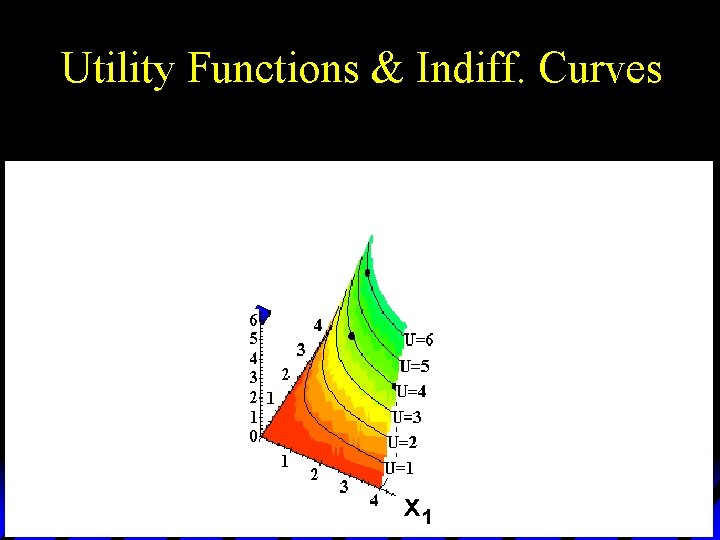Utility Functions & Indiff. Curves x 1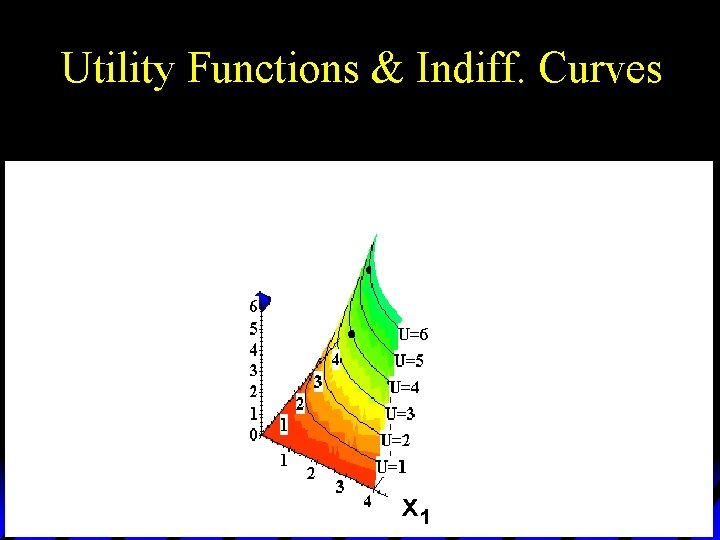Utility Functions & Indiff. Curves x 1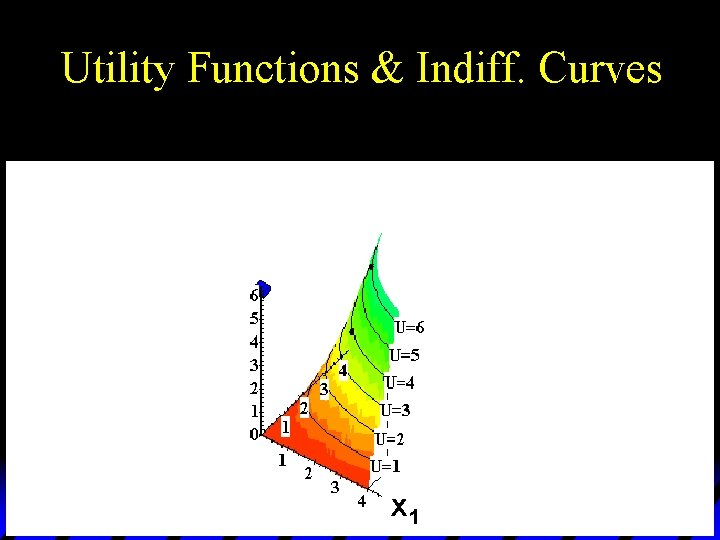Utility Functions & Indiff. Curves x 1Utility Functions & Indiff. Curves x 1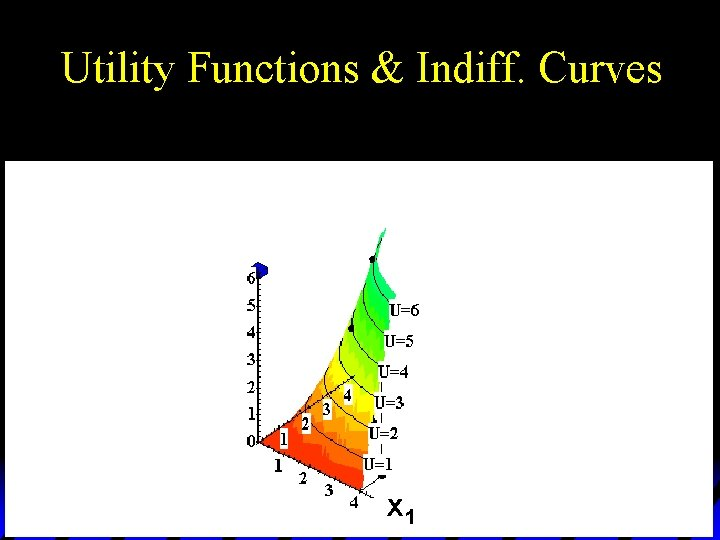Utility Functions & Indiff. Curves x 1Utility Functions & Indiff. Curves x 1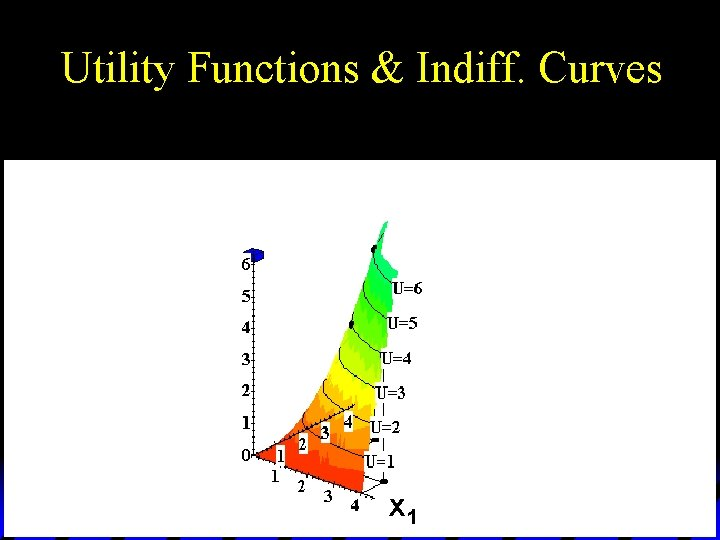Utility Functions & Indiff. Curves x 1Utility Functions & Indiff. Curves x 1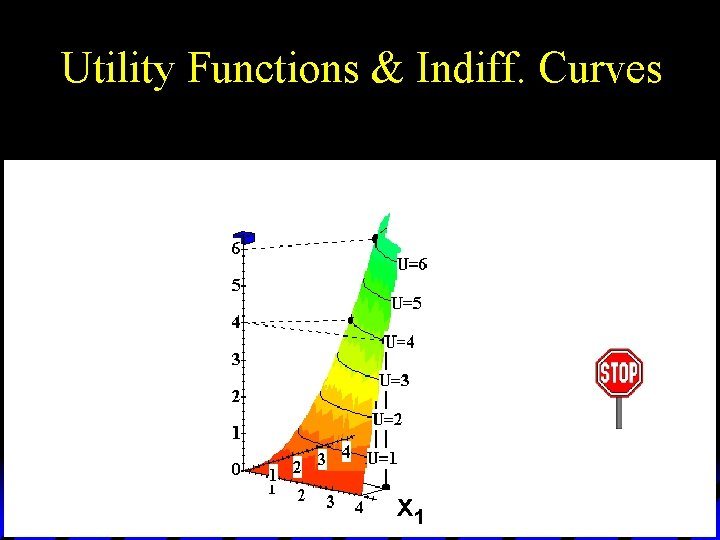Utility Functions & Indiff. Curves x 1